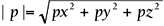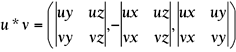# Vector Math

This section provides a quick review of vector math.

#### Modulo

The modulo of a vector can be defined as its length and is computed by:#### Dot Product

The dot product is an operation that, given two vectors, returns a floating-point value. It is usually depicted as:

` u•v `

And it is computed as follows:

` u•v=ux*vx+uy*vy+uz*vz `

Dot products are extremely popular in geometric tests. They can be computed on arbitrary vectors, but it is common to see them related to normalized (modulo-1) vectors. When applied to unit vectors, the dot product can be interpreted as the cosine of the angle between the two vectors being multiplied. Thus, the dot product of two parallel vectors equals one, and the dot product of two orthogonal (perpendicular) vectors equals zero.

#### Cross Product

The cross product is an operation that, given two vectors, returns a third vector. It is usually expressed as:

` u * v `

And is computed as follows:The members between vertical braces are the determinants of those matrices. I will define determinants in a second. For now, suffice it to say that the preceding equation can be expressed as:

` u*v=(uy*vz-uz*vy,uz*vx-ux*vz,ux*vy-uy*vx) `

which can be easily understood. But notice how cross products are more expensive to compute than the dot version. Their geometric interpretation is, however, at least as interesting. Given two unit vectors, the cross product returns a third vector that is perpendicular to the plane formed by the two initial vectors.Core Techniques and Algorithms in Game Programming2003
ISBN: N/A
EAN: N/A
Year: 2004
Pages: 261